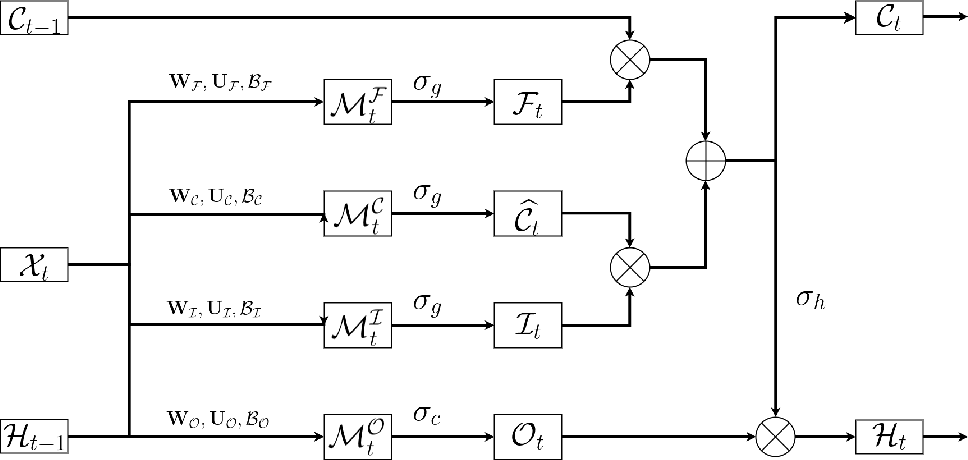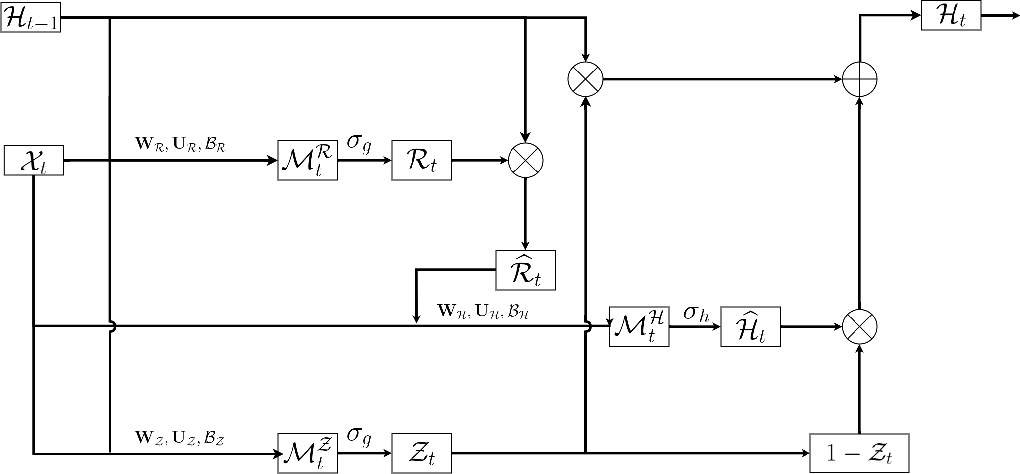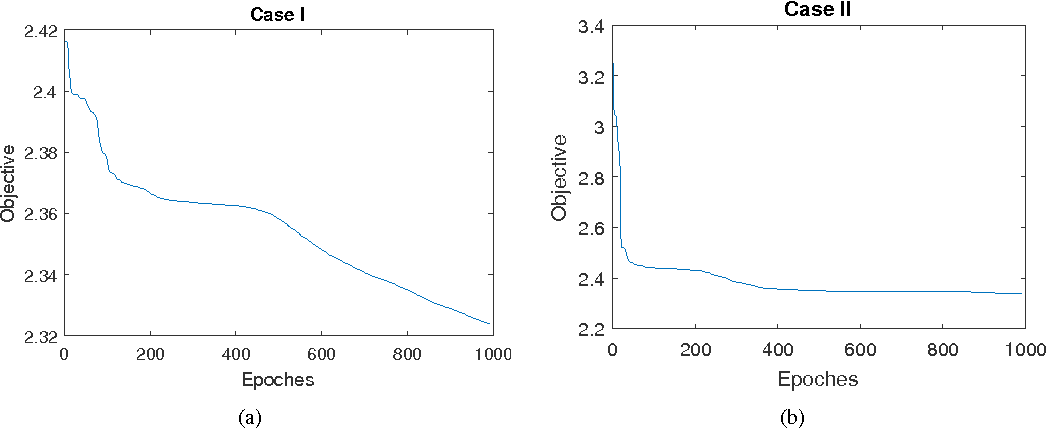Traditional Recurrent Neural Networks assume vectorized data as inputs. However many data from modern science and technology come in certain structures such as tensorial time series data. To apply the recurrent neural networks for this type of data, a vectorisation process is necessary, while such a vectorisation leads to the loss of the precise information of the spatial or longitudinal dimensions. In addition, such a vectorized data is not an optimum solution for learning the representation of the longitudinal data. In this paper, we propose a new variant of tensorial neural networks which directly take tensorial time series data as inputs. We call this new variant as Tensorial Recurrent Neural Network (TRNN). The proposed TRNN is based on tensor Tucker decomposition.Click To Get Code For Paper